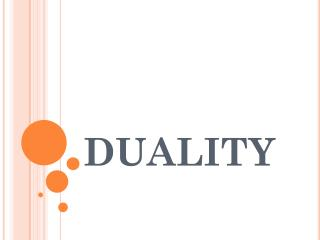DownloadDownload PresentationDUALITY

# DUALITY

Download Presentation## DUALITY

- - - - - - - - - - - - - - - - - - - - - - - - - - - E N D - - - - - - - - - - - - - - - - - - - - - - - - - - -
##### Presentation Transcript

1. DUALITY

2. Duality • Duality is the relationship between the primal and its dual, both on a mathematical and economic level, that is truly the essence of duality theory. • This theory was first developed in relation to linear programming, but it has many applications, and perhaps even a more natural and intuitive interpretation, in several related areas such as nonlinear programming, networks and game theory.

3. CONCEPT OF PRIMAL AND DUAL DUAL - A problem form obtained by transposing the rows and columns of the constraints coefficients, transposing the coefficients of the objective function, reversing the inequalities and the objective function (minimizing instead of maximizing and vice versa) of a linear programming problem. PRIMAL – The original Linear Programming Problem is known as Primal.

4. Standard form of the Primal Problem

5. Standard form of the Dual Problem

6. RULES FOR CONSTRUCTING THE DUAL PROBLEMS • If the objective of the one problem is to maximize, the objective of the other is to minimize. • The maximization problem should have all ≤ constraints and minimization problems should have ≥ constraints. If in a maximization case any constraint is ≥ type, it can be multiplied by ─1 and signs can be got reversed. Similarly if in a minimization case any problem has got ≤ type, it can be multiplied by ─1 and signs can be got reversed. • The number of Primal decision variables (xi) equals the number of dual constraints and the number of primal constraints equals the number of dual variables (yi) i.e. each dual variable corresponds to one constraint in the primal and vice versa.

7. Therefore if the primal problem has “m” constraints and “n” variables then dual problem will have “n” constraints and “m” variables. Such condition is usually violated in case of = type constraints, and should be resolved before proceeding to the solution. • If the inequalities are ≤ type, then in dual problem, they are ≥ type and vice versa. • The Cj of the primal in the objective function appears as RHS constants of the constraints, and the primal RHS constants (bi) appears as unit contribution rate i.e. Cj of the dual decision variable in the objective function. The matrix of the constraint co-efficient for one problem is the transpose of the matrix of constraints co-efficient of the other problem i.e. rows in the primal becomes columns in the dual.

8. PRIMAL – DUAL RELATIONSHIP PRIMAL DUAL • Maximization • No. of variables • No. of constraints • ≤ type constraints • = unrestricted variables • RHS constant for jth constraint • The objective function co-efficient for jth variable • Co-efficient (aij)for jth variable in the ith constraint. • Minimization • No. of constraints • No. of variables • Non-negative variable • = type constraint • Objective function co-efficient for ith variable • RHS constants for its constraints • Co-efficient (aij)for ith variable in the jth constraint.

9. INTERPRETING THE PRIMAL DUAL RELATIONSHIP • Locate the slack/surplus variables in the dual (or primal) problem. These variables corresponds to the primal (or dual) basic variable in the optimal solution. • The values in the index row corresponding to the columns of the slack/surplus variables with change in the sign gives directly the optimal values of the primal basic variables. • Value for slack/surplus variables of the primal are given by the index row under the non – basic variables of the dual solution with change in sign. • The value of the objective function is same for primal and dual problem.

10. PRIMAL DUAL Max Z = 50 x1 + 120 x2 Subject to 2 x1 + 4 x2 ≤ 80 3 x1 + x2 ≤ 60 x1, x2 ≥ 0 Min Z = 80 y1 + 60 y2 Subject to 2 y1 + 3 y2 ≥ 50 4 y1 + 1y2 ≥ 120 y1, y2 ≥ 0

11. DUAL OF THE DUAL IS PRIMAL